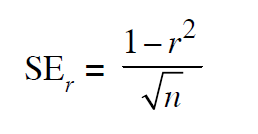# PREVIOUS YEAR SOLVED PAPERS - UGC NET Management January 2017

>>>>>>UGC NET Management January 2017

• A

A ∪ B• B

A ∩ B• C

Ā ∩ B• D

(A ∩ B)∪(Ā ∩ B)• Option : A
• Explanation : If A and B are two events, then
A ∪ B = an event which represents the occurrence of either A or B or both.
A ∩ B = an event which represents the simultaneous occurrence of A and B.
A = complement of event A and represents non-occurrence of A.
A ∩ B = both A and B do not occur.
A ∩ B = event A does not occur but event B occurs.
A ∩ B = event A occurs but event B does not occur.
(A∩B)∪(A∩B) = exactly one of the two events A and B occurs.

• Option : C
• Explanation : Bernoulli process: A process in which each trial has only two possible outcomes, the probability of the outcome at any trial remains fixed over time, and the trials are statistically independent.

• A

Both the Statements are correct.• B

Both the Statements are incorrect.• C

Statement–I is correct while Statement–II is incorrect.• D

Statement–I is incorrect while Statement– II is correct.• A

Both the Statements are correct.• B

Both the Statements are incorrect.• C

Statement–I is correct while Statement–II is incorrect.• D

Statement–I is incorrect while Statement–II is correct.• Option : A
• Explanation : Probable Error and Standard Error of Coefficient of Correlation
The probable error (PE) of coefficient of correlation indicates extent to which its value depends on the condition of random sampling. If r is the calculated value of correlation coefficient in a sample of n pairs of observations, then the standard error SEr of the correlation coefficient r is given byThe probable error of the coefficient of correlation is calculated by the expression:
PEr = 0.6745
Thus, with the help of PEr we can determine the range within which population coefficient of correlation is expected to fall using following formula:
p = r ± PEr
Where p (rho) represents population coefficient of correlation.
Remarks
1. If r < PEr then the value of r is not significant, that is, there is no relationship between two variables of interest.
2. If r > 6PEr then value of r is significant, that is, there exists a relationship between two variables.
Normality and the Sampling Distribution of the Mean
1. For most population distributions, regardless of shape, the sampling distribution of the mean is approximately normally distributed if samples of at least 30 are selected.
2. If the population distribution is fairly symmetrical, the sampling distribution of the mean is approximately normal for samples as small as five.
3. If the population is normally distributed, the sampling distribution of the mean is normally distributed, regardless of the sample size.

• A

Open• B

Expensive• C

Global• D

Untrusted